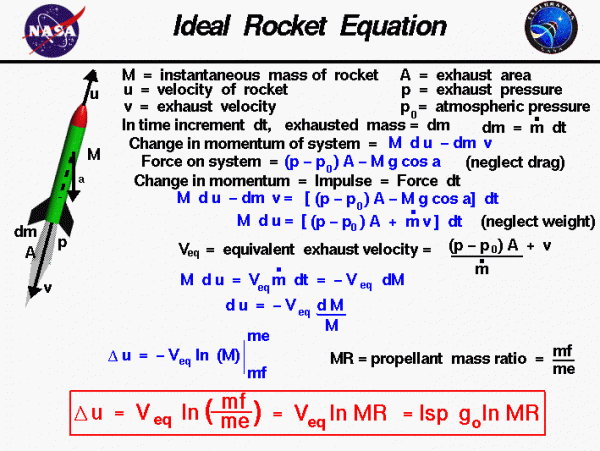# Ideal Rocket EquationThe forces on a rocket change dramatically during a typical flight. During powered flight, the propellants of the propulsion system are constantly being exhausted from the nozzle. As a result, the weight and mass of the rocket is constantly changing. Because of the changing mass, we cannot use the standard form of Newton’s second law of motion to determine the acceleration and velocity of the rocket. This figure shows a derivation of the change in velocity during powered flight while accounting for the changing mass of the rocket. In this derivation, we are going to neglect the effects of aerodynamic lift and drag. We can add these effects to the final answer.

Let us begin by considering the rocket drawing on the left of the figure. M is the instantaneous mass of the rocket, u is the velocity of the rocket, v is the velocity of the exhaust from the rocket, A is the area of the exhaust nozzle, p is the exhaust pressure and p0 is the atmospheric pressure. During a small amount of time dt a small amount of mass dm is exhausted from the rocket. This changes the mass of the rocket and the velocity of the rocket, and we can evaluate the change in momentum of the rocket as:

$$\LARGE \text{change in rocket momentum}=M(u+\text{d}u)-Mu=M\text{d}u$$

We can also determine the change in momentum of the small mass dm that is exhausted at velocity v as:

$$\LARGE \text{change in exhaust momentum}=\text{d}m(u-v)-u\text{d}m=-v\text{d}m$$

So, the total change in momentum of the system (rocket + exhaust) is:

$$\LARGE \text{change in system momentum}=M\text{d}u-v\text{d}m$$

Now consider the forces acting on the system, neglecting the drag on rocket. The weight of the rocket is M g (gravitational constant) acting at an angle a to the flight path. The pressure force is given by A(p – p0) acting in the positive u direction. Then the total force on the system is:

$$\LARGE \text{force on the system}=A(p-p_{0})-Mg\cos a$$

The change in momentum of the system is equal to the impulse on the system which is equal to the force on the system times the change in time dt. So, we can combine the previous two equations:

$$\LARGE M\text{d}_u-v\text{d}m=[A(p-p_{0})-Mg\cos a]\text{d}t$$

If we ignore the weight force, and perform a little algebra, this becomes:

$$\LARGE M\text{d}u=[A(p-p_{0})]\text{d}t+v\text{d}m$$

Now the exhaust mass dm is equal to the mass flow rate mdot ($$\bf \dot{m}$$) times the increment of time dt. So, we can write the last equation as:

$$\LARGE M\text{d}u=[A(p-p_{0})+\dot{m}v]\text{d}t$$

On the web page describing the specific impulse, we introduce the equivalent exit velocity Veq which is defined as:

$$\LARGE V_{eq}=\frac{v+A(p-p_0)}{\dot{m}}$$

If we substitute the value of Veq into the momentum equation we have:

$$\LARGE M\text{d}u=V_{eq}\dot{m}\text{d}t$$

The $$\bf \dot{m}\text{d}t$$ is the amount of change of the instantaneous mass of the rocket. The sign of this term is negative because the rocket is losing mass as the propellants are exhausted.

$$\LARGE \dot{m}\text{d}t=-\text{d}M$$

Substituting into the momentum equation:

$$\LARGE M\text{d}u=-V_{eq}\text{d}M$$

$$\LARGE \text{d}u=\frac{-V_{eq}\text{d}M}{M}$$

We can now integrate this equation:

$$\LARGE \Delta u=-V_{eq}\ln M$$

where delta u ($$\bf \Delta u$$) represents the change in velocity, and ln is the symbol for the natural logarithmic function. The limits of integration are from the initial mass of the rocket to the final mass of the rocket. The instantaneous mass of the rocket M, the mass is composed of two main parts, the empty mass me and the propellant mass mp.

The empty mass does not change with time, but the mass of propellants on board the rocket does change with time:

$$\LARGE M(t)=m_{e}+m_{p}(t)$$

Initially, the full mass of the rocket mf contains the empty mass and all of the propellant at lift off. At the end of the burn, the mass of the rocket contains only the empty mass:

$$\LARGE M_{initial}=m_{f}=m_{e}+m_{p}$$

$$\LARGE M_{final}=m_{e}$$

Substituting for these values we obtain:

$$\LARGE \Delta u=V_{eq}\ln(\frac{m_{f}}{m_{e}})$$

This equation is called the ideal rocket equation. There are several additional forms of this equation which we list here:

Using the definition of the propellant mass ratio MR

$$\LARGE \mathit{MR}=\frac{m_{f}}{m_{e}}$$

$$\LARGE \Delta u=V_{eq}\ln(\mathit{MR})$$

Veq is related to the specific impulse Isp:

$$\LARGE V_{eq}=\mathit{Isp}\cdot g_{0}$$

where g0 is the gravitational constant. So the change in velocity can be written in terms of the specific impulse of the engine:

$$\LARGE \Delta u=\mathit{Isp}\cdot g_{0}\cdot\ln(\mathit{MR})$$

If we have a desired delta u for a maneuver, we can invert this equation to determine the amount of propellant required:

$$\LARGE \mathit{MR}=e^{(\frac{\Delta u}{\mathit{Isp}\cdot g_{0}})}$$

where e is the exponential function.

What good is all this math? Let’s check the results of the equation with a few numbers. From our discussion of specific impulse and as shown in the thrust simulator, a reasonable value for specific impulse for a liquid hydrogen/liquid oxygen rocket (like the Space Shuttle main engine) would be about 350 seconds. The delta u needed to get to a 200 mile high orbit is given by the circular orbit simulator as about 17,000 mph (~25,000 feet/sec). The gravitational constant for Earth is 32.2 feet/second squared.

Putting these values into the ideal rocket equation, the resulting mass flow ratio MR is equal to 10. From the ideal rocket equation, 90% of the weight of a rocket going to orbit is propellant weight. The remaining 10% of the weight includes structure, engines, and payload. So given the current state-of-the-art, the payload accounts for only about 1% of the weight of an ideal rocket at launch. Rockets are terribly inefficient and expensive.

If you include the effects of gravity in the rocket equation, the equation becomes:

$$\LARGE \Delta u=V_{eq}\ln(\mathit{MR})-g_{0}\cdot\mathit{tb}$$

where tb is the time for the burn.

Provide feedback